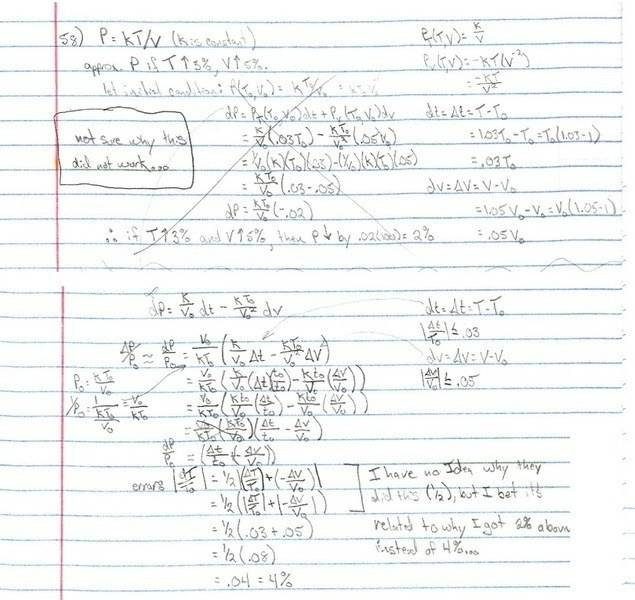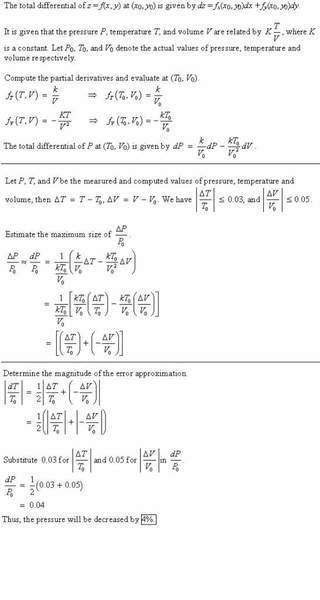# Ideal Gas Law - Differential Approximation due to variable increase in %.

• Beamsbox

## Homework Statement

This is a problem form my calculus book, which states:

According to the ideal gas law, the pressure, temperature, and volume of a confined gas are related by P=kT/V, where K is a constant. Use differentials to approximate the percentage change in pressure if the temperature of a gas is increased 3% and the volume is increased 5%.

## The Attempt at a Solution

Here, I include my original attempt at the solution and a solution that I found online. In my original solution, I found the answer to be 2%, but the solution says 4%. I'm not sure why... but I think it's related to something I don't understand in the actual solution, where it seems to me, they divide by 1/2 for no apparent logical reason.

Could someone please explain to me where my logic is flawed?Here is a solution I found online to the same problem, perhaps it's more clear.#### Attachments

Last edited:
Edited to make images easier to see. (Sorry.)